In this week’s blog we will follow up the previous series of espresso thermal analysis with a thermal analysis that is a little more fitting for this time of the year. As summer drags on and the days get warmer, everyone’s primary mission is to stay cool. One of the most common methods for this is a nice cold drink. A drink becomes cold when it gives energy to a colder system, such as ice. Thermal energy is always transferred from the warmer system to the colder system until an equilibrium point is reached. This means that ice cannot infinitely cool water; there is always a limit to how cold the water can get and this limit is known as the freezing point (0°C, 32°F).

## Heat Transfer Experiment

Purpose

The purpose of this experiment is to understand the impact that ice has on the thermal energy of a glass cup of water.

Scope

The scope of this experiment is to use a glass cup of water and cubes of ice to find the cooling relationship between them. The glass cup will be covered to nullify any losses to evaporation or convection. Each temperature will be measured using an Omega™ HH806AU J-type therm°Couple reader. The glass cup will start at room temperature and then be monitored as it cools until the ice is completely melted.

Procedure

The basic procedure for this experiment will be as follows:

• Fill the glass cup with 7 ounces of room temperature water.
• Place a predetermined amount of ice cubes in the glass cup along with the therm°Couple reader and cover.
• Record temperature until ice is completely melted.

## Experimental Results of Water and Ice Heat Transfer

• Each ice cube had an approximate size of 1 ¼” x ¾” x ¾”, with a density of 0.9167g/cm3 and a measured volume of 0.5 oz. Each ice cube weighed 13.55 g.
• With one ice cube the water reached a low temperature of 19.6°C and the ice cube took 5 minutes and 51 seconds to melt.  The temperature of the water over time can be seen below in Figure 1.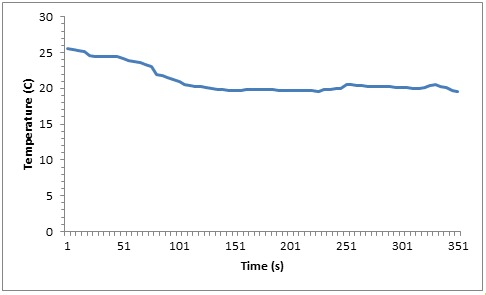Figure 1: Temperature of Water with 1 Ice Cube

• With two ice cubes the water reached a low temperature of 14.2°C and the ice cubes took 8 minutes and 16 seconds to melt. The temperature of the water over time can be seen below in Figure 2.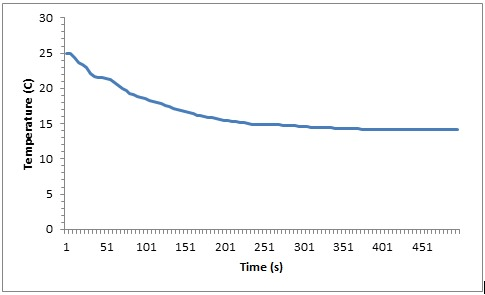Figure 2: Temperature of Water with 2 Ice Cubes

• With three ice cubes the water reached a low temperature of 10.3°C and the ice cubes took 12 minutes and 56 seconds to melt. The temperature of the water over time can be seen below in Figure 3.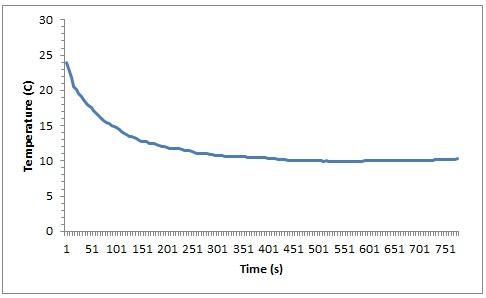Figure 3: Temperature of Water with 3 Ice Cubes

• With four ice cubes the water reached a low temperature of 6.5°C and the ice cubes took 15 minutes and 1 second to melt. The temperature of the water over time can be seen below in Figure 4.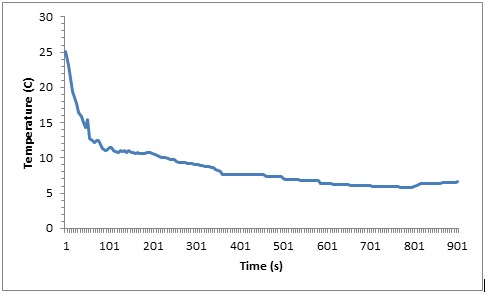Figure 4: Temperature of Water with 4 Ice Cubes

• With five ice cubes the water reached a low temperature of 5.1°C and the ice cubes took 18 minutes and 11 seconds to melt. The temperature of the water over time can be seen below in Figure 5.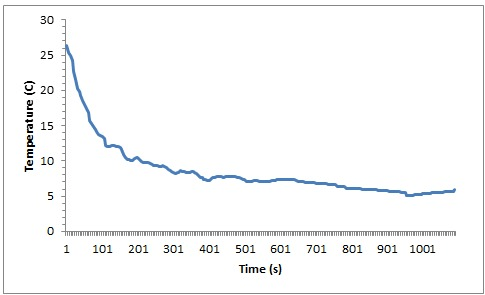Figure 5: Temperature of Water with 5 Ice Cubes

• With six ice cubes the water reached a low temperature of 4.3°C and the ice cubes took 21 minutes and 36 seconds to melt. The temperature of the water over time can be seen below in Figure 6.Figure 6: Temperature of Water with 6 Ice Cubes

• With seven ice cubes the water reached a low temperature of 4.1°C and the ice cubes took 53 minutes and 26 seconds to melt. The temperature of the water over time can be seen below in Figure 7.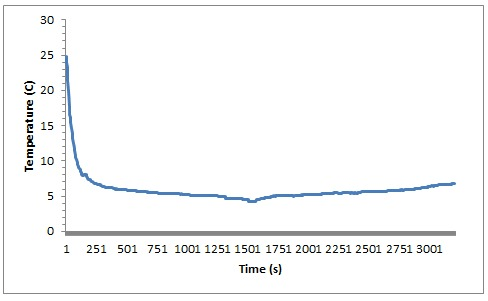Figure 7: Temperature of Water with 7 Ice Cubes

• The distribution of of the time it took to melt the ice can be seen below in Figure 8.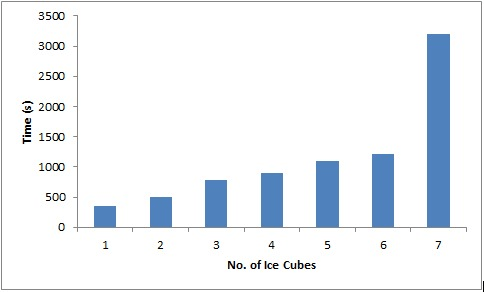Figure 8: Time Elapsed When Ice was Completely Melted

## Analysis of Water and Ice Heat Transfer

The water with just one ice cube had a theoretical low temperature of 18.5°C. This was found using Equation 1, seen below.

mice*cice*ΔTice+nice*H+mice*cwater*ΔTmeltedice+mwater*cwater*ΔTwater=0

where m is mass, c is the specific heat, T is temperature, n is the molar amount and H is the molar heat of fusion. Using the same equation the theoretical temperatures for the water with 2 through 7 ice cubes was also calculated and these values are seen in Figure 9 below.

Figure 9: Theoretical Final Temperature per Ice Cube

[table id=ice9 /]

However this theoretical temperature was not reached in the measurements due mainly to energy losses that could not be avoided. The measured temperatures can be seen in Figure 10 below.

Figure 10: Measured Final Temperature per Ice Cube

[table id=ice10 /]

The comparison between the theoretical temperatures and the measured temperatures can be seen below in Figure 11.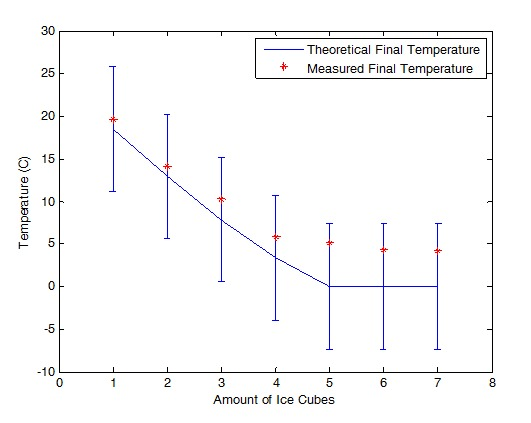Figure 11: Comparison of Theoretical and Measured Final Water Temperatures

While the measured temperature does not match exactly with the theoretical calculations, all of the measured points are within the allowable error and follow the same trend. The main losses were through free convection to the air through the walls of the glass cup and conduction to the walls of the class cup. The Biot number is a dimensionless number which is the ratio of the resistance to heat transfer on the inside and outside of the glass cup and is calculated using Equation 2 below

Bi=(h*Lc)/k                       Equation 2

where h is the heat transfer coefficient, Lc is the characteristic length and k is the thermal conductivity. The glass cup used in these experiments has a very high Biot number (606) meaning that there is a large temperature gradient between the inside of the glass cup and the outside of the glass cup. These differences can be seen in Figure 12 below.

Figure 12: Inner and Outer Surface Temperatures of Glass Cup

[table id=ice12 /]

## Conclusions

The common misconception with iced drinks is the more ice, the better. This experiment proved that this is in fact not true. This final temperatures of the water with 4, 5, 6 or 7 ice cubes were all within 1.6°C. Physically speaking, the water can only be cooled to a certain temperature, so it makes sense that the limit was consistent regardless of the amount of ice. After reaching the cooling limit the ice will affect the time at which the water stays that temperature. When comparing the temperature of the water with 6 ice cubes against the water with 7 ice cubes, their minimum temperatures are only 0.1°C off. However it took the 7 ice cubes 31 minutes and 50 seconds longer to melt completely and 6 ice cubes only took 21 minutes and 36 seconds.

In order to use the least amount of ice for the coldest drink the results point to the 4 ice cube sample. This doesn’t mean that you should always use just four ice cubes in your drink, it means that you want about 20% of your drink (by volume) to be ice. A good rule of thumb would be approximately 1 ice cube per every five ounces of beverage.